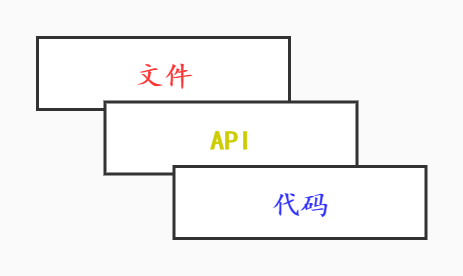# Python进阶教程m7d–混合编程–代码级扩展Python扩展的3个层级

### 2.构建pyd文件的步骤

#### 2.1 step1、编写C/C++函数

``````#include <python.h>//包含python.h
int add(int a,int b)
{
return a + b;
}``````

#### 2.2 step2、封装函数

``````PyObject* wrap_add_i(PyObject* self, PyObject* args)
{
//定义入参、返回值变量
int a,b, result;

//解析参数，解析的结果赋值到变量a和b
if (!PyArg_ParseTuple(args, "ii", &a,&b))//第2个字符串参数声明C语言函数的入参类型，第1个i表示被封装函数第1个入参为int型，依次类推
return NULL;

//调用被封装函数
result = add(a,b);

//返回结果
return Py_BuildValue("i", result);//第1个字符串参数声明返回变量的类型，i表示int型
}``````

#### 2.3 step3、构建PyMethodDef 数据

``````struct PyMethodDef {
const char  *ml_name;   /* The name of the built-in function/method */
PyCFunction ml_meth;    /* The C function that implements it */
int         ml_flags;   /* Combination of METH_xxx flags, which mostly
describe the args expected by the C func */
const char  *ml_doc;    /* The __doc__ attribute, or NULL */
};
typedef struct PyMethodDef PyMethodDef;``````

ml_name是导出函数的名称，这个才是最终给Python调用的函数名称，是字符串类型，ml_meth是被封装函数的函数名称，ml_flags是标志位，ml_doc是函数说明字符串可以为NULL。

``````static PyMethodDef  MethodsDef[] =
{
//导出函数名称, 封装函数，参数模式，函数说明
{ "add_i",  wrap_add_i,  METH_VARARGS, "Caculate two int"},

{ NULL, NULL, NULL, NULL }
};``````

#### 2.4 step4、构造PyModuleDef数据

PyModuleDef也是一个结构体数据，第2个成员变量是要导出的模块名称，字符串变量，第5个成员变量是一个PyMethodDef *指针，填入用前面第3步定义的 MethodsDef ，其他的变量可以用如下默认参数。

``````static PyModuleDef ModuleDef = {
PyModuleDef_HEAD_INIT,
"c2pyd",   //导出的模块名称
NULL,      //模块文档，可以为NULL
-1,       //一般为-1
MethodsDef //PyMethodDef类型，导出方法
};``````

#### 2.5 step5、定义 PyInit_xyz()函数

``````PyMODINIT_FUNC
PyInit_c2pyd(void)
{
return PyModule_Create(&ModuleDef);
}``````

### 3.使用模块

``````import c2pyd  #不需要pyd后缀
print('c2pyd.add_i(1,5)=',c2pyd.add_i(1,5),'\n') #add_i在static PyMethodDef  MethodsDef[]中定义的``````
``````==========结果:
c2pyd.add_i(1,5)= 6``````

### 4.添加更多函数

``````float add(float a, float b)
{
return a + b;
}``````

``````PyObject* wrap_add_f(PyObject* self, PyObject* args)
{
float a, b, result;
if (!PyArg_ParseTuple(args, "ff", &a, &b))//解析为2个float类型
return NULL;
result = add(a, b);
return Py_BuildValue("f", result);//返回float型结果
}``````

``````static PyMethodDef  MethodsDef[] =
{
//导出函数名称, 封装函数，参数模式，函数说明
{ "add_i",  wrap_add_i,  METH_VARARGS, "Caculate two int"},
{ "add_f",  wrap_add_f,  METH_VARARGS, "Caculate two float" },//添加
{ NULL, NULL, NULL, NULL }
};``````

``````print('-----欢迎来到www.juzicode.com')
print('-----公众号: 桔子code/juzicode \n')
import c2pyd  #不需要pyd后缀
print('c2pyd.add_i(1,5)=',c2pyd.add_i(1,5),'\n') #add_i在static PyMethodDef  MethodsDef[]中定义的
print('c2pyd.add_f(1.1,5.5)=',c2pyd.add_f(1.1,5.5),'\n')``````
``````==========结果:
c2pyd.add_i(1,5)= 6

c2pyd.add_f(1.1,5.5)= 6.599999904632568 ``````

### 5.其他

#### 5.1 PyMethodDef最后一个元素

``````static PyMethodDef  MethodsDef[] =
{
//导出函数名称, 封装函数，参数模式，函数说明
{ "add_i",  wrap_add_i,  METH_VARARGS, "Caculate two int"},
{ "add_f",  wrap_add_f,  METH_VARARGS, "Caculate two float" },
//{ NULL, NULL, NULL, NULL }  //
};``````
``````print('-----欢迎来到www.juzicode.com')
print('-----公众号: 桔子code/juzicode \n')

print('-----导入模块')
import c2pyd  #不需要pyd后缀
print('-----调用函数前')
print('c2pyd.add_i(1,5)=',c2pyd.add_i(1,5),'\n') #add_i在static PyMethodDef  MethodsDef[]中定义的
print('c2pyd.add_f(1.1,5.5)=',c2pyd.add_f(1.1,5.5),'\n')
print('-----调用函数后')``````

#### 5.2 返回多个值

``````PyObject* wrap_add_sub_f(PyObject* self, PyObject* args)
{
float a, b, result, result2;
if (!PyArg_ParseTuple(args, "ff", &a, &b))//解析为2个float类型
return NULL;
result = add(a, b);
result2 = sub(a, b);
return Py_BuildValue("ff", result, result2);//返回float型结果的和以及差值
}

static PyMethodDef  MethodsDef[] =
{
//导出函数名称, 封装函数，参数模式，函数说明
{ "add_i",  wrap_add_i,  METH_VARARGS, "Caculate two int"},
{ "add_f",  wrap_add_f,  METH_VARARGS, "Caculate two float" },
{ "add_sub_f",  wrap_add_sub_f,  METH_VARARGS, "Caculate two float" },
{ NULL, NULL, NULL, NULL }
};``````
``````import c2pyd  #不需要pyd后缀

print('c2pyd.add_i(1,5)=',c2pyd.add_i(1,5),'\n') #add_i在static PyMethodDef  MethodsDef[]中定义的
print('c2pyd.add_f(1.1,5.5)=',c2pyd.add_f(1.1,5.5),'\n')
print('c2pyd.add_sub_f(1.1,5.5)=',c2pyd.add_sub_f(1.1,5.5),'\n')``````
``````==========结果:
c2pyd.add_i(1,5)= 6

c2pyd.add_f(1.1,5.5)= 6.599999904632568

c2pyd.add_sub_f(1.1,5.5)= (6.599999904632568, -4.400000095367432)``````

add_sub_f()函数在C代码的封装函数中返回的多个值，在Python的调用后返回的是一个tuple。

#### 5.3 返回None

``````PyObject* wrap_do_nothing(PyObject* self, PyObject* args)
{
return Py_BuildValue("");//返回float型结果的和以及差值
}
static PyMethodDef  MethodsDef[] =
{
//导出函数名称, 封装函数，参数模式，函数说明
{ "add_i",  wrap_add_i,  METH_VARARGS, "Caculate two int"},
{ "add_f",  wrap_add_f,  METH_VARARGS, "Caculate two float" },
{ "add_sub_f",  wrap_add_sub_f,  METH_VARARGS, "Caculate two float" },
{ "ret_nothing",  wrap_ret_nothing,  METH_VARARGS, "ret nothing" },
{ NULL, NULL, NULL, NULL }
};``````
``````import c2pyd  #不需要pyd后缀

print('c2pyd.ret_nothing(1.1,5.5)=',c2pyd.ret_nothing(1.1,5.5),'\n')``````
``````==========结果：
c2pyd.ret_nothing(1.1,5.5)= None``````

#### 5.4 没有入参

``````
PyObject* wrap_trans_noargs(PyObject* self, PyObject* args)
{
printf("%s","wrap_trans_noargs: nothing\n");

return Py_BuildValue("");//返回float型结果的和以及差值
}

static PyMethodDef  MethodsDef[] =
{
//导出函数名称, 封装函数，参数模式，函数说明
{ "add_i",  wrap_add_i,  METH_VARARGS, "Caculate two int"},
{ "add_f",  wrap_add_f,  METH_VARARGS, "Caculate two float" },
{ "add_sub_f",  wrap_add_sub_f,  METH_VARARGS, "Caculate two float" },
{ "ret_nothing",  wrap_ret_nothing,  METH_VARARGS, "ret nothing" },
{ "trans_noargs",  wrap_trans_noargs,  METH_NOARGS, "transfer noargs" },

{ NULL, NULL, NULL, NULL }
};``````
``````import c2pyd
print('c2pyd.trans_noargs()=',c2pyd.trans_noargs(),'\n')``````
``````==========结果：
trans_noargs: nothing     #这里是c函数内部的打印内容
c2pyd.trans_noargs()= None``````

#### 5.4 关键字入参

``````PyObject* wrap_trans_kwargs(PyObject* self, PyObject* args,PyObject *kw)
{
char* kwlist[] = { "a","b","c","d", NULL };//最后一个元素必须为NULL，NULL前面的元素名称就是python调用传入的参数名称
float a, b, c,d;
if (!PyArg_ParseTupleAndKeywords( args, kw, "ffff", kwlist,  &a, &b, &c, &d))
return NULL;
printf("wrap_trans_kwargs: a=%f, b=%f, c=%f, d=%f\n", a,b,c,d);
float result = add(a, b)+ add(c, d);

return Py_BuildValue("f",result);//返回float型结果的和以及差值
}

static PyMethodDef  MethodsDef[] =
{
//导出函数名称, 封装函数，参数模式，函数说明
{ "add_i",  wrap_add_i,  METH_VARARGS, "Caculate two int"},
{ "add_f",  wrap_add_f,  METH_VARARGS, "Caculate two float" },
{ "add_sub_f",  wrap_add_sub_f,  METH_VARARGS, "Caculate two float" },
{ "ret_nothing",  wrap_ret_nothing,  METH_VARARGS, "ret nothing" },
{ "trans_noargs",  wrap_trans_noargs,  METH_NOARGS, "transfer  noargs" },
{ "trans_kwargs",  (PyCFunction)wrap_trans_kwargs, METH_VARARGS| METH_KEYWORDS, "transfer kwargs" },

{ NULL, NULL, NULL, NULL }
};``````
``````import c2pyd
print('c2pyd.trans_kwargs(1.1,3.3,5.5,7.7)=',c2pyd.trans_kwargs(1.1,3.3,5.5,7.7),'\n')
print('c2pyd.trans_kwargs(1.1,3.3,c=5.5,d=7.7)=',c2pyd.trans_kwargs(1.1,3.3,c=5.5,d=7.7),'\n')
print('c2pyd.trans_kwargs(a=1.1,b=3.3,c=5.5,d=7.7)=',c2pyd.trans_kwargs(a=1.1,b=3.3,c=5.5,d=7.7),'\n') ``````
``````==========结果：
wrap_trans_kwargs: a=1.100000, b=3.300000, c=5.500000, d=7.700000
c2pyd.trans_kwargs(1.1,3.3,5.5,7.7)= 17.600000381469727

wrap_trans_kwargs: a=1.100000, b=3.300000, c=5.500000, d=7.700000
c2pyd.trans_kwargs(1.1,3.3,c=5.5,d=7.7)= 17.600000381469727

wrap_trans_kwargs: a=1.100000, b=3.300000, c=5.500000, d=7.700000
c2pyd.trans_kwargs(a=1.1,b=3.3,c=5.5,d=7.7)= 17.600000381469727``````